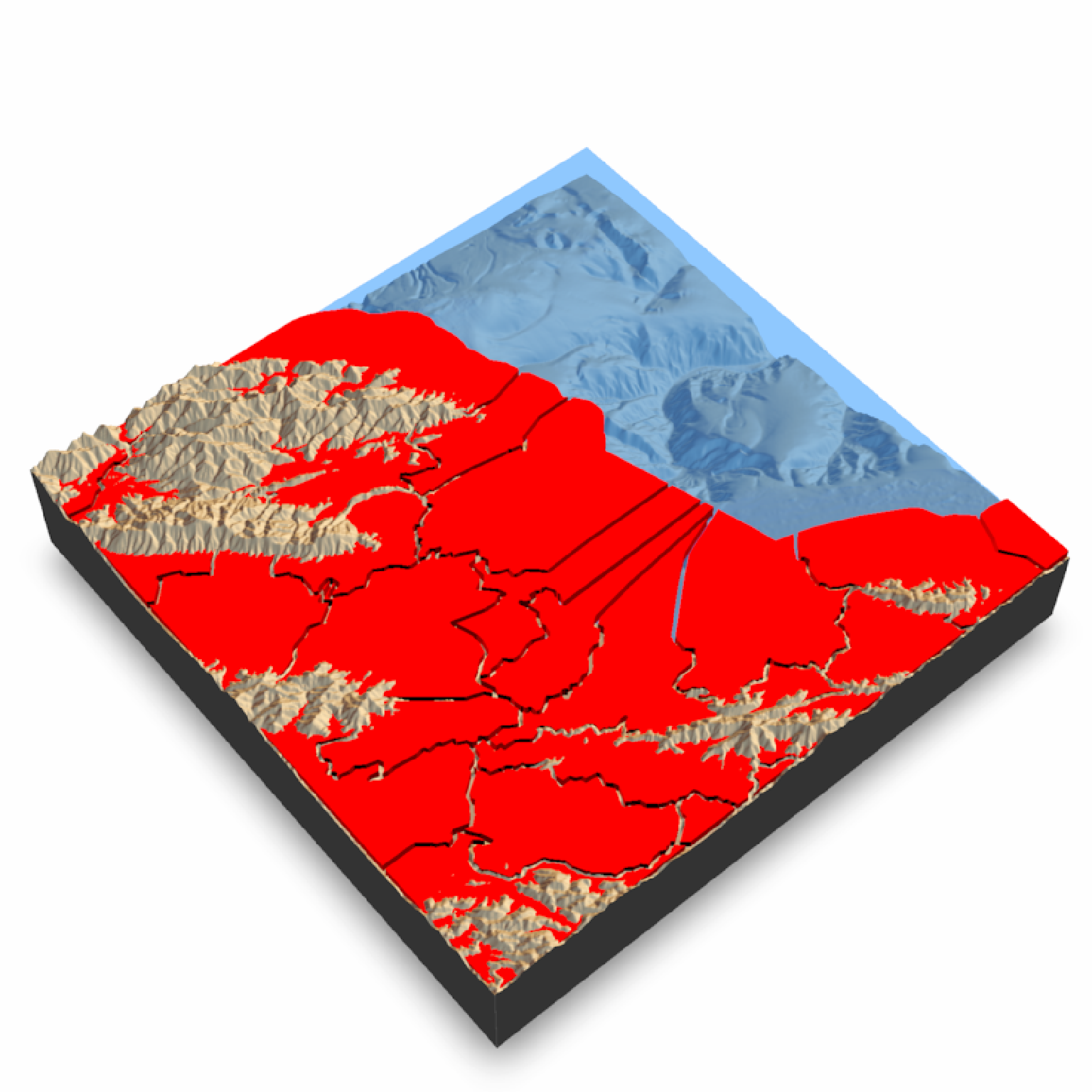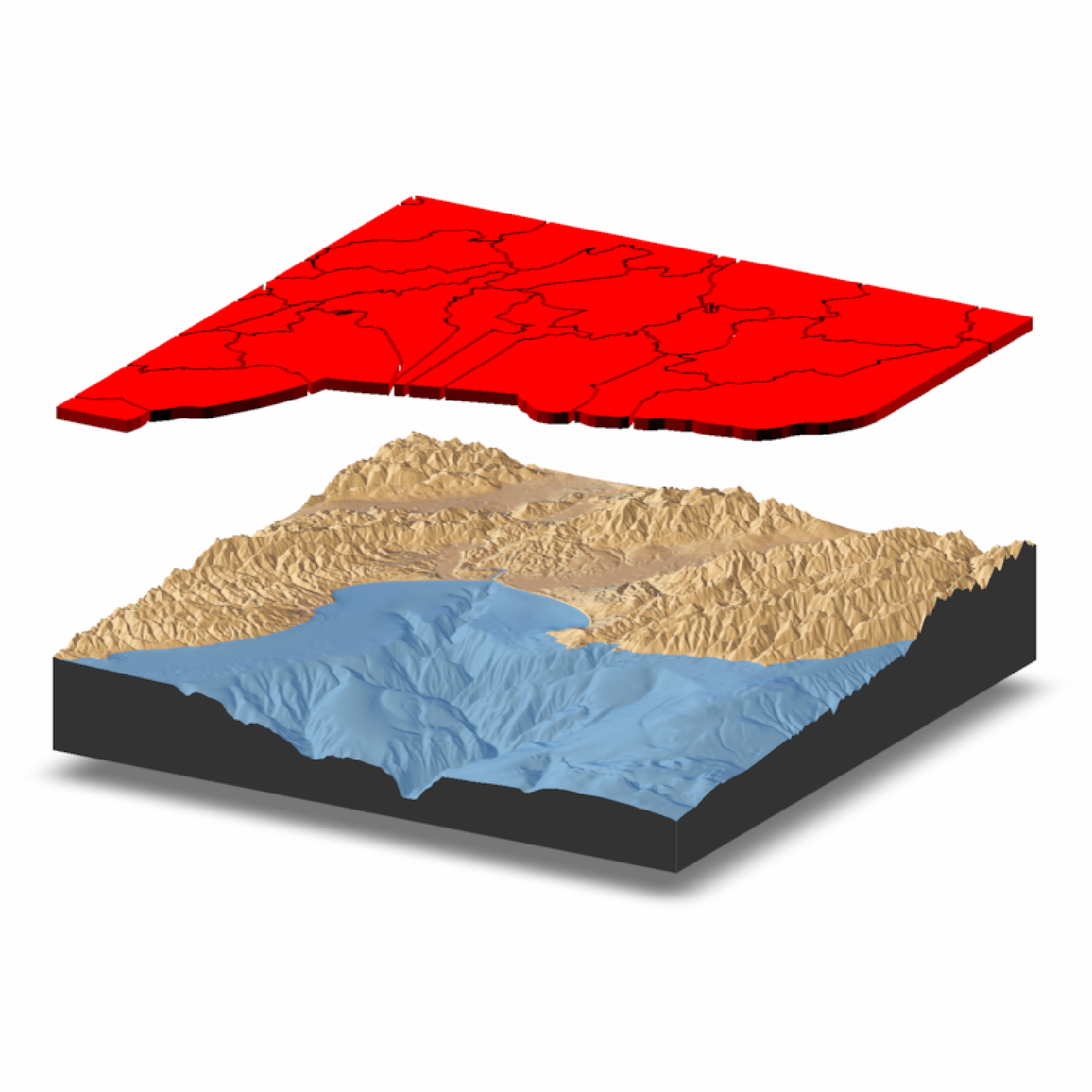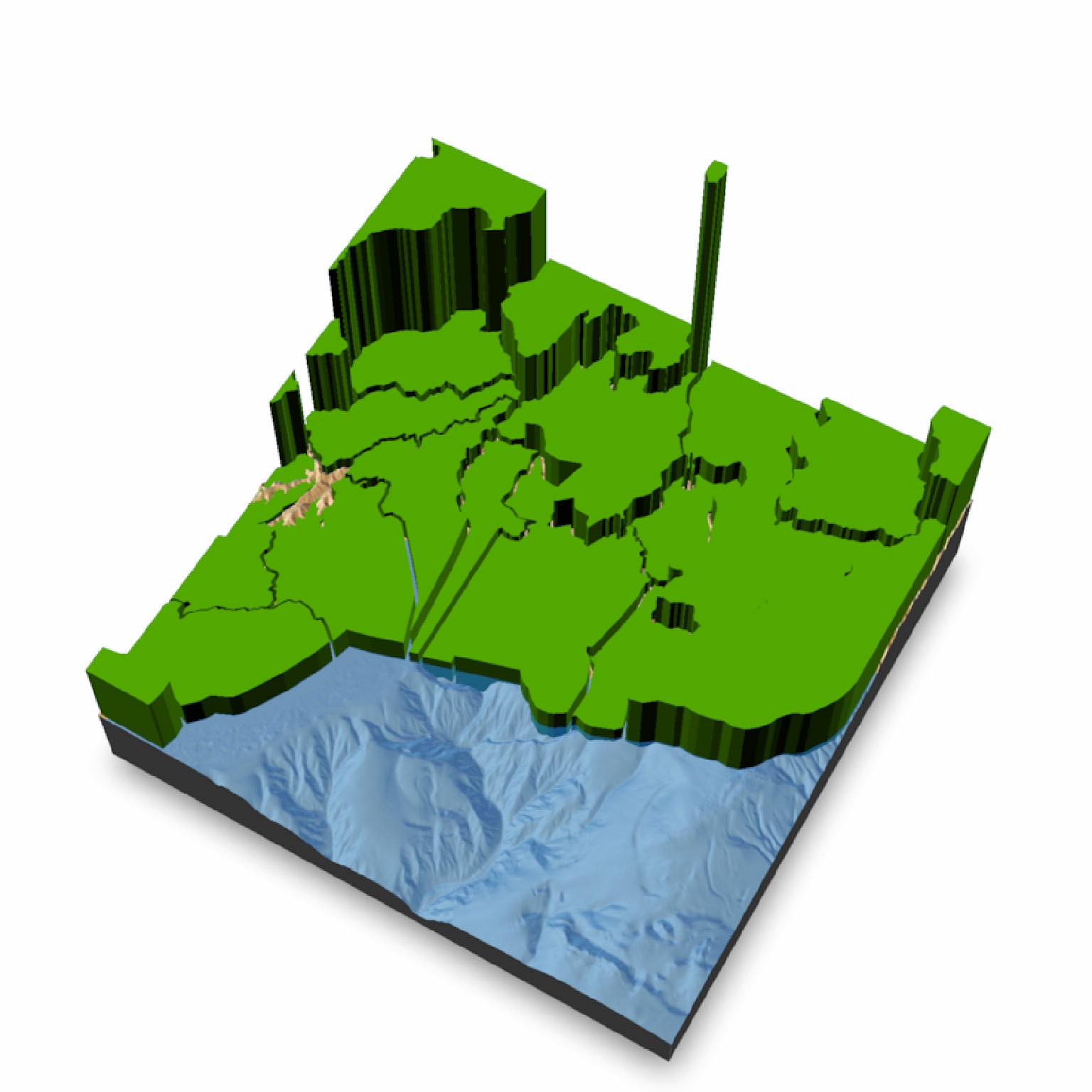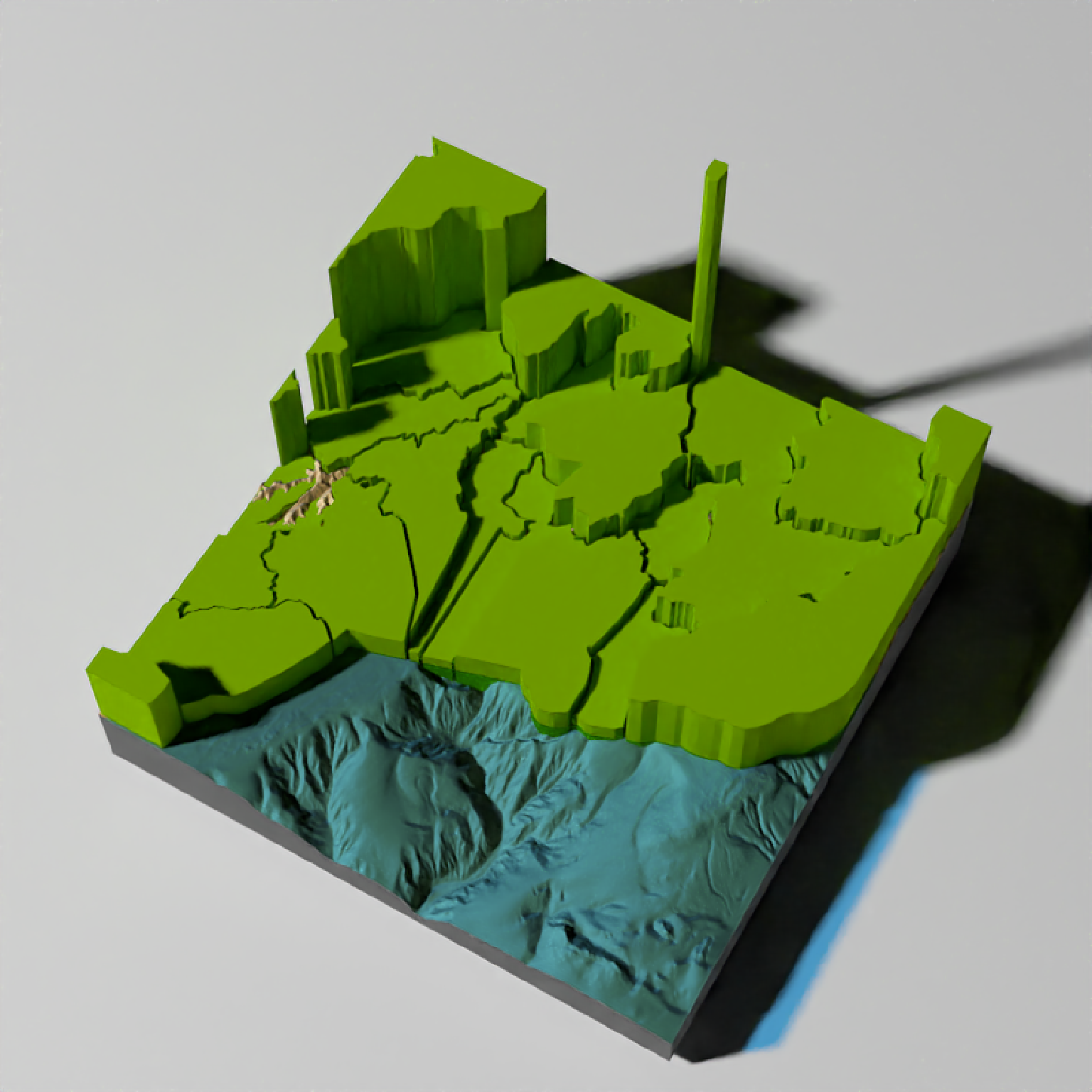Adds 3D polygons to the current scene, using latitude/longitude or coordinates in the reference system defined by the extent object.

render_polygons(
polygon,
extent,
color = "red",
top = 1,
bottom = NA,
data_column_top = NULL,
data_column_bottom = NULL,
heightmap = NULL,
scale_data = 1,
parallel = FALSE,
holes = 0,
alpha = 1,
lit = TRUE,
light_altitude = c(45, 30),
light_direction = c(315, 135),
light_intensity = 0.3,
clear_previous = FALSE
)

## Arguments

polygon

sf object, "SpatialPolygon" sp object, or xy coordinates of polygon represented in a way that can be processed by xy.coords(). If xy-coordinate based polygons are open, they will be closed by adding an edge from the last point to the first.

extent

Either an object representing the spatial extent of the 3D scene (either from the raster, terra, sf, or sp packages), a length-4 numeric vector specifying c("xmin", "xmax", "ymin", "ymax"), or the spatial object (from the previously aforementioned packages) which will be automatically converted to an extent object.

color

Default black. Color of the polygon.

top

Default 1. Extruded top distance. If this equals bottom, the polygon will not be extruded and just the one side will be rendered.

bottom

Default 0. Extruded bottom distance. If this equals top, the polygon will not be extruded and just the one side will be rendered.

data_column_top

Default NULL. A string indicating the column in the sf object to use to specify the top of the extruded polygon.

data_column_bottom

Default NULL. A string indicating the column in the sf object to use to specify the bottom of the extruded polygon.

heightmap

Default NULL. Automatically extracted from the rgl window--only use if auto-extraction of matrix extent isn't working. A two-dimensional matrix, where each entry in the matrix is the elevation at that point. All points are assumed to be evenly spaced.

scale_data

Default 1. If specifying data_column_top or data_column_bottom, how much to scale that value when rendering.

parallel

Default FALSE. If TRUE, polygons will be extruded in parallel, which may be faster (depending on how many geometries are in polygon).

holes

Default 0. If passing in a polygon directly, this specifies which index represents the holes in the polygon. See the earcut function in the decido package for more information.

alpha

Default 1. Transparency of the polygons.

lit

Default TRUE. Whether to light the polygons.

light_altitude

Default c(45, 60). Degree(s) from the horizon from which to light the polygons.

light_direction

Default c(45, 60). Degree(s) from north from which to light the polygons.

light_intensity

Default 0.3. Intensity of the specular highlight on the polygons.

clear_previous

Default FALSE. If TRUE, it will clear all existing polygons.

## Examples

if(run_documentation()) {
#Render the county borders as polygons in Monterey Bay
montereybay %>%
plot_3d(montereybay, water = TRUE, windowsize = 800, watercolor = "dodgerblue")
render_camera(theta = 140,  phi = 55, zoom = 0.85, fov = 30)

#We will apply a negative buffer to create space between adjacent polygons. You may
#have to call sf::sf_use_s2(FALSE) before running this code to get it to run.
sf::sf_use_s2(FALSE)
mont_county_buff = sf::st_simplify(sf::st_buffer(monterey_counties_sf,-0.003), dTolerance=0.001)

render_polygons(mont_county_buff,
extent = attr(montereybay,"extent"), top = 10,
parallel = TRUE)
render_snapshot()
}
#> montereybay dataset used with no zscale--setting zscale=50.  For a realistic depiction, raise zscale to 200.
#> Warning: st_buffer does not correctly buffer longitude/latitude data
#> dist is assumed to be in decimal degrees (arc_degrees).
#> Warning: st_simplify does not correctly simplify longitude/latitude data, dTolerance needs to be in decimal degreesif(run_documentation()) {
#We can specify the bottom of the polygons as well. Here I float the polygons above the surface
#by specifying the bottom argument. We clear the previous polygons with clear_previous = TRUE.
render_camera(theta=-60,  phi=20, zoom = 0.85, fov=0)
render_polygons(mont_county_buff,
extent = attr(montereybay,"extent"), bottom = 190, top=200,
parallel=TRUE,clear_previous=TRUE)
render_snapshot()
}if(run_documentation()) {
#We can set the height of the data to a column in the sf object: we'll use the land area.
#We'll have to scale this value because its max value is 2.6 billion:
render_camera(theta=-60,  phi=60, zoom = 0.85, fov=30)
render_polygons(mont_county_buff,
extent = attr(montereybay, "extent"), data_column_top = "ALAND",
scale_data = 300/(2.6E9), color = "chartreuse4",
parallel = TRUE, clear_previous = TRUE)
render_snapshot()
}if(run_documentation()) {
#This function also works with render_highquality()
render_highquality(samples = 128, clamp_value = 10, sample_method="sobol_blue",
min_variance = 0)
}